Updating search results...

# 480 Results

View
Selected filters:
• math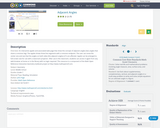Conditional Remix & Share Permitted
CC BY-NC-SA
Rating
0.0 stars

An interactive applet and associated web page that show the concept of adjacent angles (two angles that share a common leg). The applet shows three line segments with a common endpoint. The user can move the center one and see that the angles on both sides (the adjacent angles) of it are affected. Applet can be enlarged to full screen size for use with a classroom projector. After use in the classroom, students can access it again from any web browser at home or in the library with no login required. This resource is a component of the Math Open Reference Interactive Geometry textbook project at http://www.mathopenref.com.

Subject:
Mathematics
Material Type:
Simulation
Author:
John Page
08/05/2020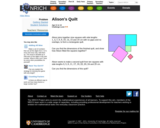Unrestricted Use
CC BY
Rating
0.0 stars

In this Nrich problem students can work on both prime factorization and area of rectangles.

Subject:
Mathematics
Material Type:
Activity/Lab
Homework/Assignment
06/18/2021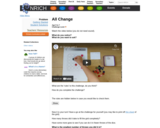Unrestricted Use
CC BY
Rating
0.0 stars

At the basic level, these Nrich challenges offer chances for children to practice number recognition, one-to-one correspondence and counting. However, some will begin to analyze and compare the three versions, explaining their findings and possibly drawing on ideas associated with probability.

Subject:
Mathematics
Material Type:
Activity/Lab
Game
Interactive
06/10/2021Conditional Remix & Share Permitted
CC BY-NC-SA
Rating
0.0 stars

SPARK tails artists Jim Denevan and Cris Drury as they create large earth works. This Educator Guide is about the history and tradition of artists making work in and about the natural environment.

Subject:
Arts and Humanities
Material Type:
Lesson Plan
Provider:
KQED Education
Provider Set:
KQED Education Network
05/18/2005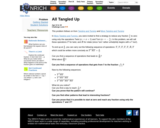Unrestricted Use
CC BY
Rating
0.0 stars

This Nrich problem follows on from Twisting and Turning and More Twisting and Turning and offers students the opportunity to explore sequences of fractions, make generalizations, and prove conjectures. The rope trick in the first problem offers a moment of surprise when the rope becomes untangled, and this prompts curious students to want to explain what is going on. This third problem in the sequence ties up all the loose ends still remaining.

Subject:
Mathematics
Material Type:
Activity/Lab
06/17/2021Unrestricted Use
CC BY
Rating
0.0 stars

This Nrich problem embeds adding and subtracting fractions into a bigger problem to increase interest.

Subject:
Mathematics
Material Type:
Activity/Lab
06/17/2021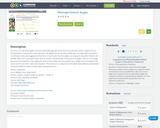Conditional Remix & Share Permitted
CC BY-NC-SA
Rating
0.0 stars

An interactive applet and associated web page that demonstrate the alternate exterior angles that are formed where a transversal crosses two lines. The applets shows the two possible pairs of angles alternating when in animation mode. By dragging the three lines, it can be seen that the angles are congruent only when the lines are parallel. When not in animated mode, there is a button that alternates the two pairs of angles. The text on the page discusses the properties of the angle pairs both in the parallel and non-parallel cases. Applet can be enlarged to full screen size for use with a classroom projector. This resource is a component of the Math Open Reference Interactive Geometry textbook project at http://www.mathopenref.com.

Subject:
Mathematics
Material Type:
Simulation
Author:
John Page
08/05/2020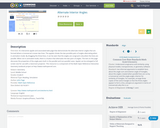Conditional Remix & Share Permitted
CC BY-NC-SA
Rating
0.0 stars

An interactive applet and associated web page that demonstrate the alternate interior angles that are formed where a transversal crosses two lines. The applets shows the two possible pairs of angles alternating when in animation mode. By dragging the three lines, it can be seen that the angles are congruent only when the lines are parallel. When not in animated mode, there is a button that alternates the two pairs of angles. The text on the page discusses the properties of the angle pairs both in the parallel and non-parallel cases. Applet can be enlarged to full screen size for use with a classroom projector. This resource is a component of the Math Open Reference Interactive Geometry textbook project at http://www.mathopenref.com.

Subject:
Mathematics
Material Type:
Simulation
Author:
John Page
08/05/2020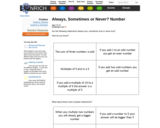Unrestricted Use
CC BY
Rating
0.0 stars

These Nrich tasks are a great opportunity for learners to use reasoning to decipher mathematical statements. We often make mathematical claims that are only true in certain contexts and it is important for learners to be able to look critically at statements and understand in what situations they apply.

Subject:
Mathematics
Material Type:
Activity/Lab
Homework/Assignment
06/18/2021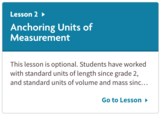Unrestricted Use
CC BY
Rating
0.0 stars

Unit 3: Unit Rates and Percentages
Lesson 2: Anchoring Units of Measurement

This lesson is optional. Students have worked with standard units of length since grade 2, and standard units of volume and mass since grade 3. This lesson is designed to anchor students’ perception of standard units of length, volume, weight, and mass with a collection of familiar objects that they can refer to in later lessons in preparation for using ratio reasoning to convert measurement units.

The main task of this lesson is a card-sorting activity in which students match common objects with their closest unit of length, volume, mass, or weight to establish anchor quantities for each unit of measurement. Since this lesson reinforces standards from previous grade levels instead of introducing grade 6 standards, if you believe that your students already have a firm grasp of these units of measurement, you may choose to skip this lesson.

Subject:
Mathematics
Ratios and Proportions
Material Type:
Activity/Lab
04/12/2021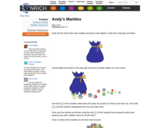Unrestricted Use
CC BY
Rating
0.0 stars

This Nrich problem involves complicated reasoning about fractions that challenges children's understandings of the concepts involved. It is a good example of how fractions relate to multiplication and division.

Subject:
Mathematics
Material Type:
Activity/Lab
06/17/2021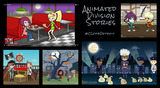Conditional Remix & Share Permitted
CC BY-NC-SA
Rating
0.0 stars

Students will work with a partner to write, solve, check,&nbsp;and animate a division story problem based on a division expression using a sharing model.

Subject:
Composition and Rhetoric
Computer Science
English Language Arts
Mathematics
Numbers and Operations
Speaking and Listening
Material Type:
Lesson Plan
Author:
Jody Walker
03/24/2019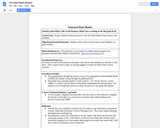Rating
0.0 stars

To give students need more practice with the relationship between factors and multiples.

Subject:
Algebra
Mathematics
Material Type:
Lesson
Provider:
Michigan Virtual
Author:
Laura Harkema
05/19/2017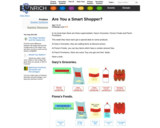Unrestricted Use
CC BY
Rating
0.0 stars

This Nrich activity is particularly good in a number of mathematical aspects of learning:
Using mathematical ideas and methods to solve "real life" problems
Using and understanding vocabulary and notation related to money
Organizing and using data
Choosing and using appropriate number operations and calculation strategies
Explaining methods and reasoning
Making and investigating general statements

Subject:
Mathematics
Material Type:
Activity/Lab
Homework/Assignment
06/18/2021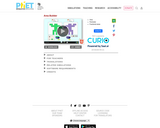Unrestricted Use
CC BY
Rating
0.0 stars

Create your own shapes using colorful blocks and explore the relationship between perimeter and area. Compare the area and perimeter of two shapes side-by-side. Challenge yourself in the game screen to build shapes or find the area of funky figures. Try to collect lots of stars!

Subject:
Mathematics
Material Type:
Simulation
Provider:
Provider Set:
PhET Interactive Simulations
Author:
Amanda McGarry
Ariel Paul
Bryce Gruneich
John Blanco
Kathy Perkins
03/20/2020Conditional Remix & Share Permitted
CC BY-NC-SA
Rating
0.0 stars

An interactive applet and associated web page that demonstrate the area of a circle. A circle is shown with a point on the circumference that can be dragged to resize the circle. As the circle is resized, the radius and the area computation is shown changing in real time. The radius and formula can be hidden for class discussion. Applet can be enlarged to full screen size for use with a classroom projector. This resource is a component of the Math Open Reference Interactive Geometry textbook project at http://www.mathopenref.com.

Subject:
Mathematics
Material Type:
Simulation
Author:
John Page
08/05/2020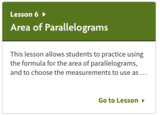Unrestricted Use
CC BY
Rating
0.0 stars

Unit: Area and Surface Area
Lesson 6: Area of Parallelograms

This lesson allows students to practice using the formula for the area of parallelograms, and to choose the measurements to use as a base and a corresponding height. Through repeated reasoning, they see that some measurements are more helpful than others. For example, if a parallelogram on a grid has a vertical side or horizontal side, both the base and height can be more easily determined if the vertical or horizontal side is used as a base.

Along the way, students see that parallelograms with the same base and the same height have the same area because the products of those two numbers are equal, even if the parallelograms look very different. This gives us a way to use given dimensions to find others.

Subject:
Geometry
Mathematics
Material Type:
Activity/Lab
04/07/2021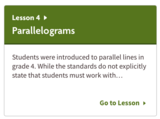Unrestricted Use
CC BY
Rating
0.0 stars

Reasoning to Find Area: Lesson 4

Students were introduced to parallel lines in grade 4. While the standards do not explicitly state that students must work with parallelograms in grades 3–5, the geometry standards in those grades invite students to learn about and explore quadrilaterals of all kinds. The K–6 Geometry Progression gives examples of the kinds of work that students can do in this domain, including work with parallelograms.

In this lesson, students analyze the defining attributes of parallelograms, observe other properties that follow from that definition, and use reasoning strategies from previous lessons to find the areas of parallelograms.

By decomposing and rearranging parallelograms into rectangles, and by enclosing a parallelogram in a rectangle and then subtracting the area of the extra regions, students begin to see that parallelograms have related rectangles that can be used to find the area.

Throughout the lesson, students encounter various parallelograms that, because of their shape, encourage the use of certain strategies. For example, some can be easily decomposed and rearranged into a rectangle. Others—such as ones that are narrow and stretched out—may encourage students to enclose them in rectangles and subtract the areas of the extra pieces (two right triangles).

After working with a series of parallelograms, students attempt to generalize (informally) the process of finding the area of any parallelogram (MP8).

Subject:
Geometry
Mathematics
Material Type:
Activity/Lab
04/06/2021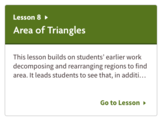Unrestricted Use
CC BY
Rating
0.0 stars

Unit: Area and Surface Area
Lesson 8: Area of Triangles

This lesson builds on students’ earlier work decomposing and rearranging regions to find area. It leads students to see that, in addition to using area-reasoning methods from previous lessons, they can use what they know to be true about parallelograms (i.e. that the area of a parallelogram is ) to reason about the area of triangles.

Students begin to see that the area of a triangle is half of the area of the parallelogram of the same height, or that it is the same as the area of a parallelogram that is half its height. They build this intuition in several ways:

by recalling that two copies of a triangle can be composed into a parallelogram;
by recognizing that a triangle can be recomposed into a parallelogram that is half the triangle’s height; or
by reasoning indirectly, using one or more rectangles with the same height as the triangle.
They apply this insight to find the area of triangles both on and off the grid.

Subject:
Geometry
Mathematics
Measurement and Data
Material Type:
Activity/Lab
04/07/2021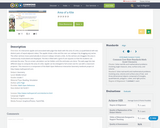Conditional Remix & Share Permitted
CC BY-NC-SA
Rating
0.0 stars

An interactive applet and associated web page that deals with the area of a kite, (a quadrilateral with two distinct pairs of equal adjacent sides). The applet shows a kite and the user can reshape it by dragging any vertex. The other vertices move automatically to ensure it always remains a kite. As the vertices are dragged, the area is continuously recalculated and displayed. The kite is filled with a grid of unit squares so that the students can estimate the area. The on-screen calculation can be hidden until the estimates are done. The web page lists two different ways to compute the area of a kite. Applet can be enlarged to full screen size for use with a classroom projector. This resource is a component of the Math Open Reference Interactive Geometry textbook project at http://www.mathopenref.com.

Subject:
Mathematics
Material Type: